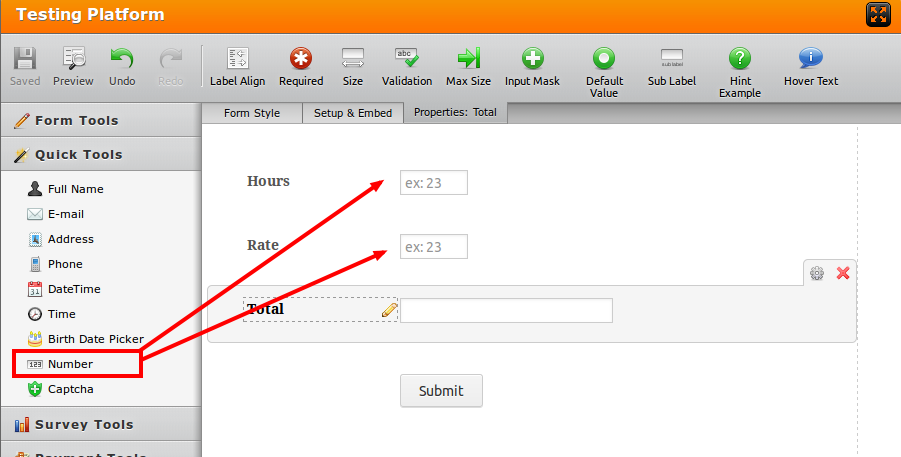# Form Calculation Issue: Decimal Points

•nolitahouse
Asked on January 18, 2014 at 11:51 AM

I am at trying to us the calculation feature to multiply a number times a percentage.  I get the correct numerical value but there are no decimal points.  I am not directly adding the percentage amount into the forumula [{tipAmount}*0.33], but rather taking a percentage calculation made from another field Example [{tipAmount}*{tipPercentageCalc}].  I don't seem to have a problem when doing the former, multiplying a field times a percentage number, but I don't get any decimal points when doing the latter.

The URL below is to the form...I am trying to get tip percentages based on the number of hours each employee works.  I am getting the percentages, but when I am trying to multiple those percentages by the tip amounts the field does not calculate the decimal points.  Eg. if you put each of the 3 runners down for 10 hours worked and put the cash tips and credit tips at \$100 each, you will see that it accurage calculates the split to 33%.  But when you try and multiple that 33% by the cash tip or credit tip \$100 I get 3300 instead of 33.00.

Thanks,

•TitusN
Answered on January 18, 2014 at 05:07 PM

Hello,

Thank you for reporting this.

Please replace all the calculation fields and the fields where you are sourcing the calculation values with the number field under quick tools as illustrated below:This happens because the calculation wizard will not treat your figures as integers but as whole numbers, since the fields where the data is colledted is a text field.

Please let us know if this helps.

•Answered on January 20, 2014 at 01:00 PM

I believe the user is referring to an issue that has been reported several times - JotForm calculations are not recognizing decimals.  Many of us are still waiting on a solution from the engineers.

•TitusN
Answered on January 20, 2014 at 01:05 PM

That's true, It has been reported before.

But if you isolate numerical calculations to the number fields, your result should be accurate, and the decimal point be placed where it should be.

Here, give it a try: http://jotformpro.com/form/40134731898964

•Answered on January 20, 2014 at 01:46 PM

Ahhh, I do see how changing the fields to number fields seems to offer a solution to our decimal issue, but can we hide the arrows for that field so that it appears to only accept typed values?

•Mike_T
Answered on January 20, 2014 at 02:21 PM

You can inject the following CSS code from this tutorial to remove these arrows:

input[type=number]::-webkit-inner-spin-button,
input[type=number]::-webkit-outer-spin-button {
-webkit-appearance: none;
margin: 0;
}Form example:

http://form.jotformpro.com/form/40195474230955

If you need any further assistance, please let us know.

•Answered on January 20, 2014 at 02:28 PM

You guys are so darn good! Seriously.  Thanks.

•Answered on January 20, 2014 at 03:17 PM

So, I am so close to completing a form but am stuck on one final problem.  I have a form that requests numerical input from users on several optional fields.  At the end, I have a calculation field (Grand Total) that sums all of the numerical fields.  For some reason, the Grand Total will not appear unless all of the numerical fields are completed.  Can I not just get a running total that sums just the fields the user completes?

•TitusN
Answered on January 20, 2014 at 03:24 PM

Hmmm,

Try changing this setting on the calculation wizard:If it does not work, please open a new thread and we shall work it out with you.

Thanks.

••